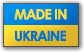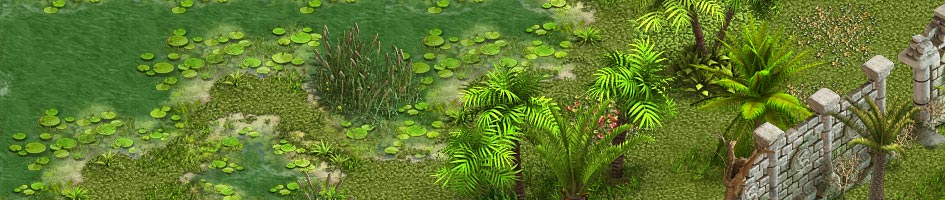# SPOILER: Keepers Village Puzzle

1 2 3 ... 5 6 7 8 9
 Reply to Amynicole The clues are helpful though really. That is a lot of extra work to do without using them. Edited 1 minute later by dbarns. 5 years ago 5 years ago
 Anonymous Квест в Keeper's Village я прошла так. Подсказки 8=4, 9=4, 3=0. Цветы 8 белых, 9 желтых, 4 красных.Таким образом, нужно вычислить разницу из подсказок. Для 8=4 - это 4, для 9=4 - это 5, для 3=0 - это 0.Вычитаем получившуюся разницу (одну из разниц) из белых 8-4=4, 9-4=5, 3-4=-1 Затем пробуем набрать на круге, перед началом надо убедиться, что кнопка не нажата . Набираем 4, жмем, набираем 5, жмем, набираем 1 жмем. Эта комбинация не подошла.Пробую с другой, 3=0, разница 3, получим 5, 6, 1. Эта комбинация сработала. 5 years ago 5 years agoReply to lucille Well i still cant figure out the puzzle, any detailed help is very welcome pleaseThere are 3 possible solutions of the puzzle, only one of them is correct. SPOILER!!!                Use this link to calculate the possible answers:or PM me, if you can't  cope with the tableI would appreciate all feedback about the result, so as to change the algorithm if necessary.Your site gives the error 404 not found 4 years ago 4 years ago
 I have tried the spreadsheet but I am unable to plug in my numbers to try and solve the puzzle (spreadsheet is in view only mode).  please please assist...my clues are: 4=1 3=2 1=9.  the flowers are 3w 8y 6r. any assistance would be greatly appreciated as this puzzle has had me scratching temple for days!!  TYIA 4 years ago 4 years ago
 It works exactly like the Zweistein Puzzle, so for 4=1 you get (+7) or (-3), which is the same result -> 053 . For 1=9 it's (+8) -> 164, for 3=2 (-1) -> 275. Always worked for me that way. 4 years ago 4 years ago
 please solve the puzzle 5=9 7=2 7=6 4 years ago 4 years ago
 Reply to rocksagar please solve the puzzle 5=9 7=2 7=6To solve it, we need to know how many bunches of white, yellow and red flowers there are around your puzzle - everyone's Keepers Village is slightly different. 4 years ago 4 years ago
 please solve the puzzle 5=9 7=2 7=6 By the way, in case you want to work it out for yourself:5=9 means add 4 (so add 4 to the number of white, yellow and red flowers to get one possible solution)7=2 means subtract 5 (so subtract 5 from the number of white, yellow and red flowers to get your second possible solution)7=6 means subtract 1 (so subtract 1 from the number of white, yellow and red flowers to get your third possible solution)One of these three results *will* give you the correct solution 4 years ago 4 years ago